Refraction of Light: Have you ever wondered that the objects placed under water appear to be in a different manner than they actually are, mostly we have observed that the things inside water will be bigger in size. For example a lemon placed in glass filled with water, a fish in a round water bowl, red color of sky during sunrise and sunset. Why are they actually looking different as they are placed in water or under a glass slab, what is responsible for these changes?

It is all due to the light that is travelling between two different mediums. Earlier you have learned about the reflection where the light returns/bounce back into the same medium, irrespective to any surface. In this topic we consider that light travels through the medium which makes the difference. As the light travels from one medium to another medium, the light ray bends. This bending phenomenon of light is known as Refraction.

### What is this medium?

What is this medium and why this change in medium is responsible for bending of light ray?

The substance surrounding a source of light through which light travels is Optical medium, we have two types of mediums: one is optically rarer medium and optically denser medium.

Optically rarer medium: The medium in which light can travel faster (lower refractive index)

Optically denser medium: The medium in which light travels at slower rate (high refractive index)

For example consider two mediums one is air (optically rarer ) and other medium as transparent glass (optically denser), when the light incident on the glass surface, it tries to pass through the glass. In this process the light when it is entering into the glass, light starts interacting with the glass molecules and these molecules starts absorbing the energy of light which results in decreasing of speed of light and seems to bend from its original path.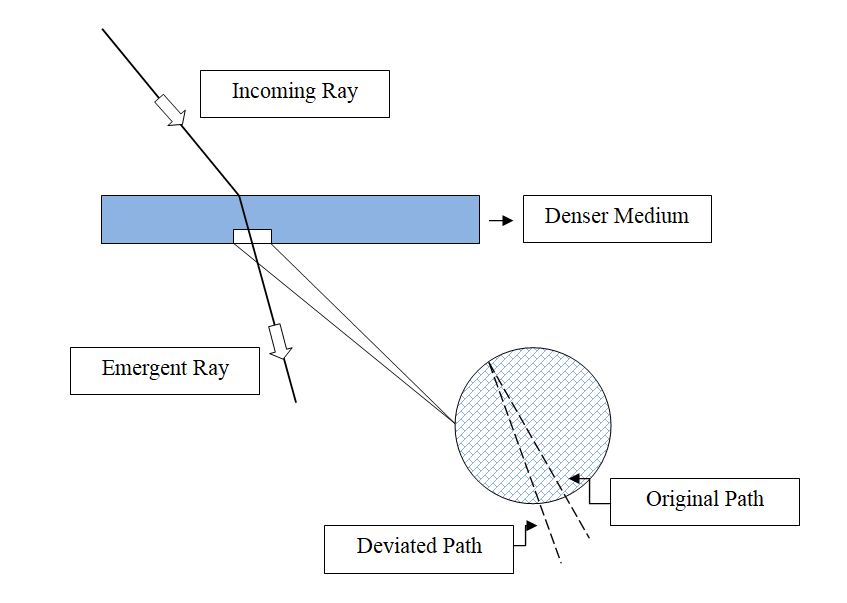As we see that light passing through the mediums it bends. How does it bend and in what direction its bends, does it always bends in one direction and can we observe that the light can bend in whatever angle the light had incident on the surface?

If a light ray incidents on a denser medium at an angle with respective to the normal, the direction of refracted ray as the light travelling from rarer medium to denser medium the light bends towards the normal as the speed of light decreases. Similarly when light travels from denser medium to rarer medium the light bends away from normal as the speed of light increases.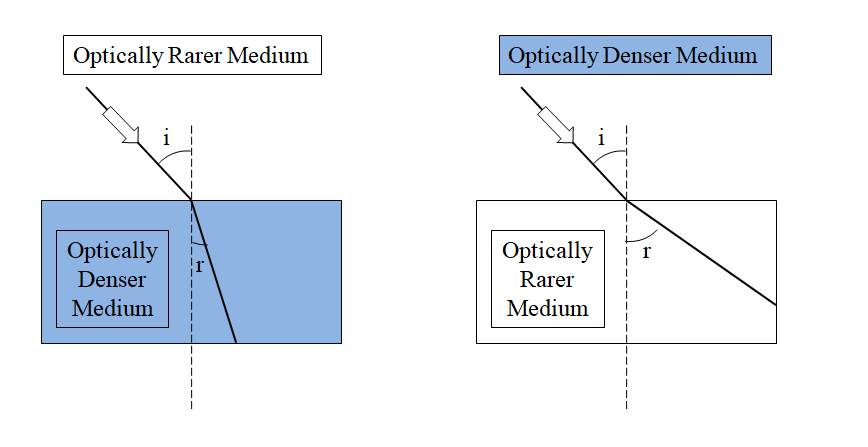In the above diagrams we can clearly see that the angle made by the incident and refracted are not same in both cases, when light travels from rarer to denser medium, the angle of incidence is greater than the angle of refraction (∟i > ∟r), similarly when the light travels from denser to rarer medium, the angle of incidence is less than the angle of refraction       (∟i < ∟r).

The deviation of light ray depends on the optical density of both the mediums. Refraction occurs only if the light incidents on the surface at an angle with respective to the normal, if a light ray incidents on the surface along the normal then the light pass in a straight line without any deviation.

### Refractive index

After knowing the direction of light, to determine till what extent the light bends from the normal is known by “Refractive Index”. It is the ratio of speed of light from rarer medium to speed of light in denser medium. It is represented by ‘µ’ or ‘n’.Refractive index is a dimensionless quantity, the refractive index ‘n’ means speed of light in that medium is the nth part of speed of light in vacuum.

If the speed of light is compared between vacuum and any medium i.e, known as absolute refractive index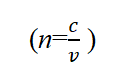If the speed of light is compared between any two different mediums (other than vacuum) it is known as relative refractive index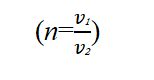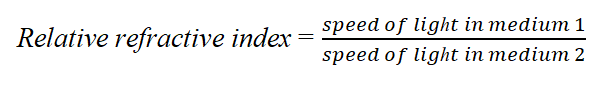In this we observe that light travels from medium 1 to medium 2 irrespective of optical density, it says that refractive index of 2nd medium is compared to the refractive index of 1st medium.Refractive index of medium 2 to the refractive index of medium 1. Refractive index depends on nature of material and wavelength of light. It can be expressed in terms of wavelengths also (if we consider light as an electromagnetic wave then velocity of wave is given by ‘v =fλ’ where v velocity of wave, f frequency of wave and λ wavelength of wave).

From this wave equation we say that velocity and wavelength are dependent of medium and where as frequency is independent of nature of medium it depends on the source alone.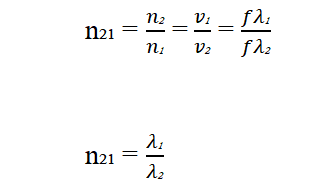### Refractive index of different materials### Laws of refraction

Ist Law: The incident ray, refracted ray and the normal to the surface separating the two mediums at the point of incidence, all lie on the same plane.

IInd Law: The ratio of sine angle of incidence to the sine angle of refraction remains constant for given pair of media and for a given color of light. This law is also known as Snell’s Law.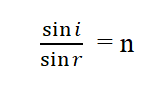(n is the refractive index and it is constant for a pair of media)

### Snell’s Law proof

Let us consider a beam of light in two cases. In first case, we observe that the light rays are incident perpendicular to the surface where all the light rays (beam) reach the surface at same time and there is no deviation in the path of light rays.In the second case, assume that the light rays are incidents with an angle, now we can see that all the light rays are not reaching the surface at the same time.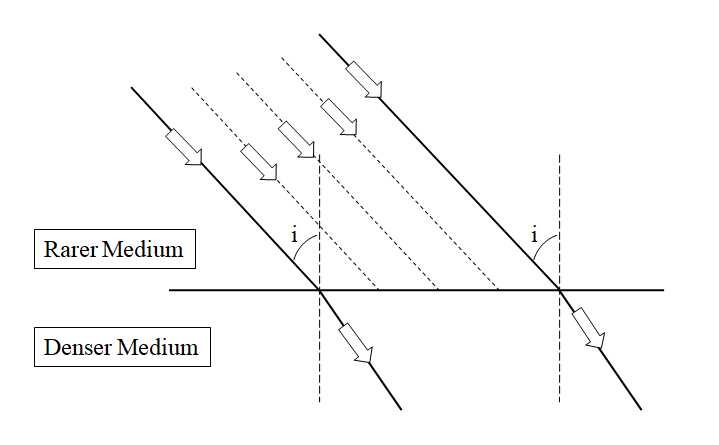It is clear from the above figure that the first ray meets the surface while the other rays are still travelling in air. Now considering the first and last ray eliminating the rays in between, as the first ray enters the second medium and gets refracted (the velocity of 1st ray decreases v2), the last ray is still travelling in the first medium with greater velocity v1 than the 1st ray. Now draw the normal ray with respective to the surface that is separating the two mediums and also draw the normal line from the 1st ray and that normal line intersects the last ray at some point.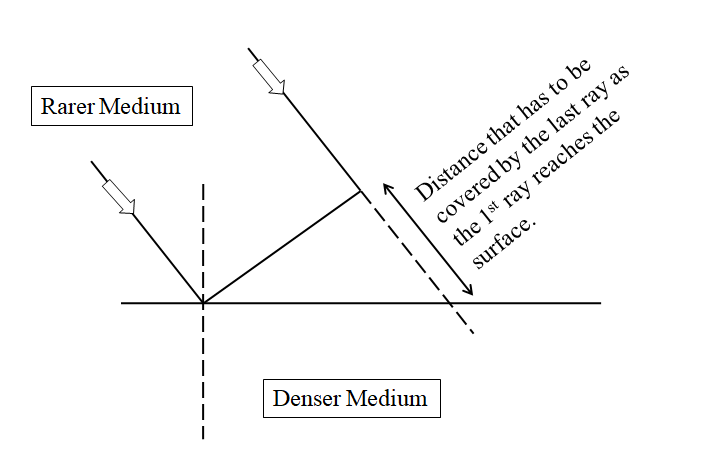Let us consider that, the time taken by the last ray is Δ‘t’ (very small time period) to reach the surface with velocity v1 , hence the displacement is given as vt. During the same time period ‘Δt’ the first ray which is in the second medium travelling with decreased velocity v2, the displacement is given as vt.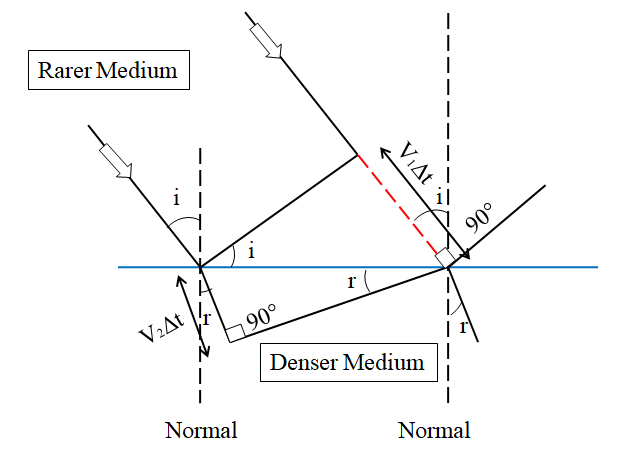In the above we have similar triangles, with a common hypotenuse we can write in the terms of sine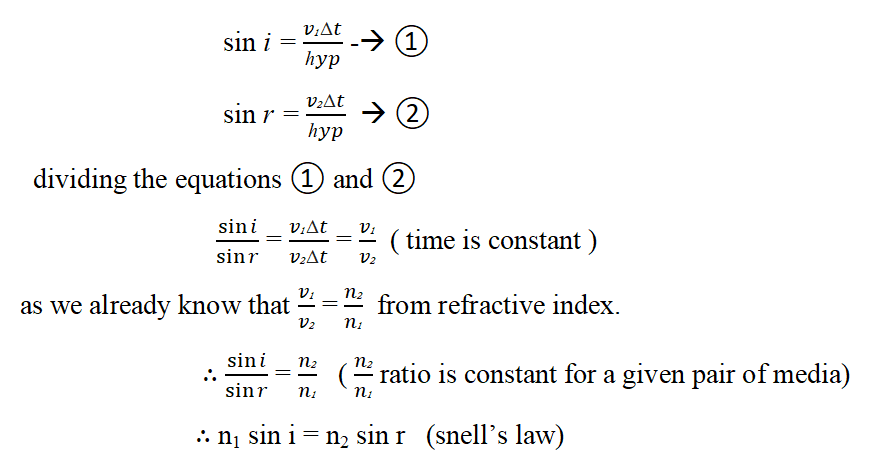If a light ray travels along the normal, no refraction takes place as angle of incidence and refraction is 0. Therefore snell’s law is not applicable when light incidents along the normal.

(sin i / sin r) = undefined, i = r = 0

### Principle of reversibility of light

If a light ray travels from medium 1 to medium 2 we observe that light ray gets refracted, and if we retrace the path of light ray from medium 2 to medium 1, the path of light is reversible this is known as Principle of reversibility of path.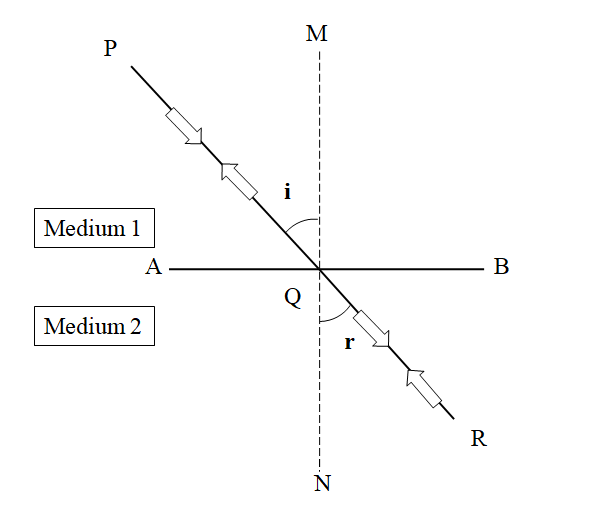Refractive index of medium 2 with respective to medium 1 is given as

similarly when the light path is retraced, refractive index of medium 1 with respective to medium 2 is given as

this equation can be written as reciprocal of n21 which is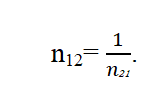### Lateral displacement

Consider a rectangular glass slab of thickness ‘t’, if a light ray incident on one of the side of glass slab with an angle i with respective to normal, the light ray normal and makes an angle r.

If there is no glass slab, the light travels in a straight line without any deviation, But due to the presence of glass slab incident ray got shifted from its original path. This shift is known as lateral displacement. The lateral displacement ‘x’ is the perpendicular distance between emergent ray and the incident ray.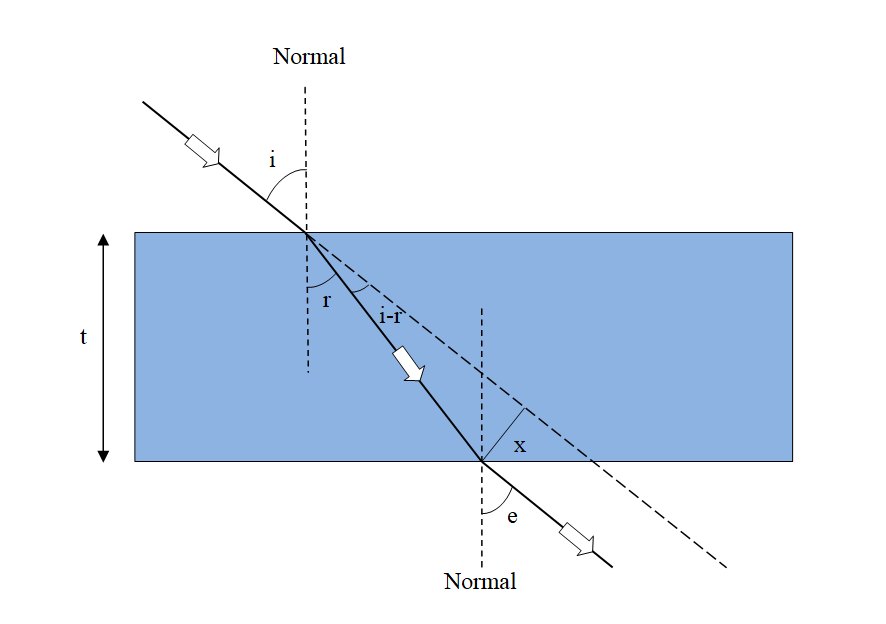The lateral displacement depends on

Thickness of medium: lateral displacement is directly proportional to thickness, as the thickness increases shift of light also increases and vice versa.

Refractive index of medium: lateral displacement is directly proportional to refractive index, as the refractive index increases shift of light also increases and vice versa.

Angle of incidence: As the angle of incidence increases lateral displacement also varies.

### Real and Apparent Depth

The things that are placed inside water or placed under glass slab seems to be raised than their actual position, and similarly when you are inside water, things seems to be far away than their actually position. This is due to refraction. Consider a empty glass tumbler and place a coin now, you can see the real position of the coin which is the real depth ‘d’.Now fill the glass tumbler with water and you will see the coin raised from its original position. This new position of the coin is the virtual image of the original object, and this height of the virtual image from the surface of the medium is apparent depth d’.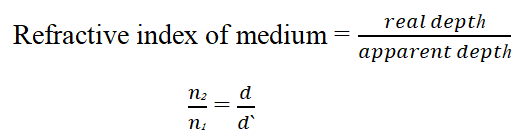Where n2 is the medium from which the light is coming and n1 is the medium where the light is going.

Both d & d’  are measured from the surface, which separates the two media.

Daily life example sun appears before it actually rises, and sun appears after the sunset.

### Total internal reflection

When a light ray passes from denser medium to rarer medium, the refracted ray bends away from the normal where the angle of refraction is greater than the angle of incidence. Gradually if we increase the angle of incidence, angle of refraction also increases. At a particular angle of incidence the refracted ray just grazes on the surface which separates the two medium by making an angle of 90⁰ with respective to the normal, this angle of incidence in denser medium is known as critical angle.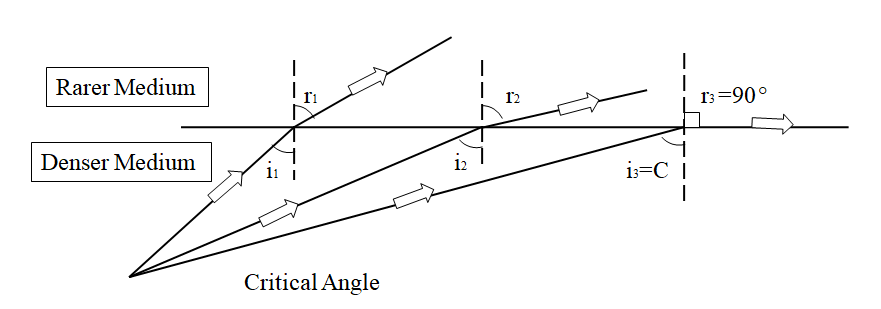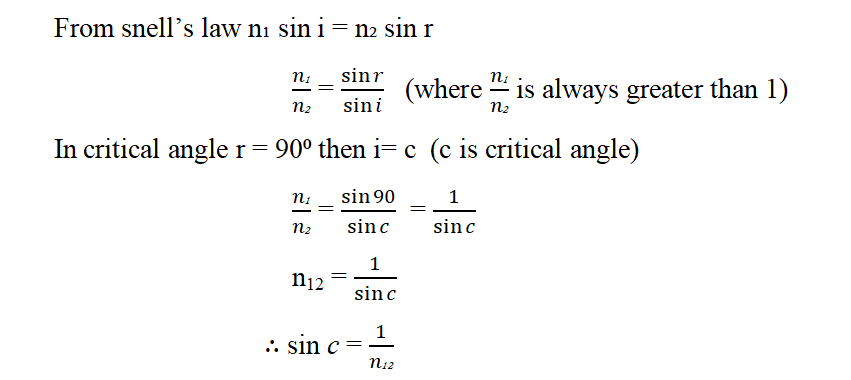If still the angle of incidence increases beyond the critical angle, the light cannot pass through the dense medium and gets reflected back into the same medium. This phenomenon of complete reflection of light into same medium is Total internal reflection.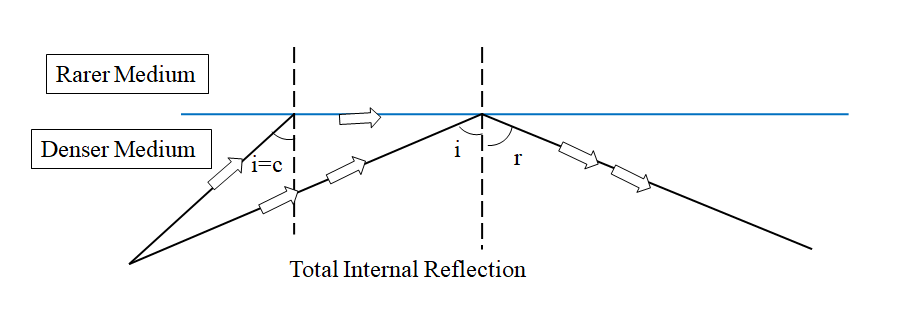### Applications of Total internal reflection

Mirage: The optical illusion of appearance of water without its actual presence is known as mirage. During a hot sunny day, the earth surface gets heated up. Due to this, a layer of air just above it gets heated and becomes less dense than the air above it. Light travelling from a distant object or a tall tree passes from high refractive index i.e., denser medium to layer of lower refractive index i.e., rare medium, which makes the light ray to refract more and more and moves away from the normal till the angle of incidence is equal to critical angle. As the angle of incidence is greater than critical angle, light gets total internal reflection and the person who observes this reflected ray sees the optical illusion of appearance of water.

Twinkling of stars: Stars appear to twinkle due to the atmospheric refraction. Light from the stars undergoes continuous refraction as it enters into the earth’s atmosphere. As the refracted ray bends towards the normal, stars appears slightly higher than their actual position. The apparent position of star changes slightly due to the change in the earth’s atmosphere, as the position of the star fluctuates. Stars appeared to twinkle.

Moon or a planet does not twinkle as it appears bigger than stars and also the moon and planets can be considered as collection of large number of point sized objects which nullify the twinkling effect.

Many more applications of total internal reflection like formation of a rainbow, diamond sparkle, optical fiber which transmits the data.